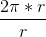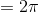# High School Math : How to find the ratio of diameter and circumference

## Example Questions

### Example Question #1 : How To Find The Ratio Of Diameter And Circumference

What is the ratio of the diameter of a circle to the circumference of the same circle?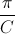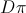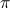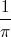Explanation:

To find the ratio we must know the equation for the circumference of a circle. In this equation,is the circumference andis the diameter.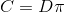Once we know the equation, we can solve for the ratio of the diameter to circumference by solving the equation for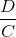. We do this by dividing both sides by.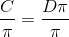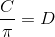Then we divide both sides by the circumference.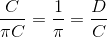We now know that the ratio of the diameter to circumference is equal to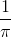.

### Example Question #2 : How To Find The Ratio Of Diameter And Circumference

What is the ratio of the diameter and circumference of a circle?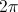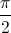Explanation:

To find the ratio we must know the equation for the circumference of a circle is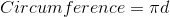Once we know the equation we can solve for the ratio of the diameter to circumference by solving the equation for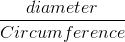we divide both sides by the circumference giving us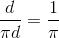We now know that the ratio of the diameter to circumference is equal to.

### Example Question #1 : How To Find The Ratio Of Diameter And Circumference

Letrepresent the area of a circle andrepresent its circumference. Which of the following equations expressesin terms of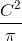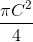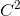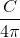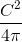Explanation:

The formula for the area of a circle is, and the formula for circumference is. If we solve for C in terms of r, we get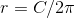.

We can then substitute this value of r into the formula for the area: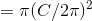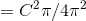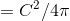### Example Question #4 : How To Find The Ratio Of Diameter And Circumference

What is the ratio of any circle's circumference to its radius?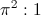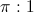Undefined.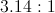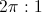Explanation:

The circumference of any circle is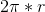So the ratio of its circumference to its radius r, is